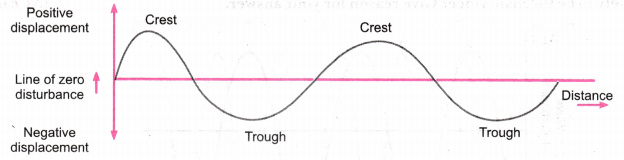# . Plot the following: A longitudinal wave in air on a density-distance graph. A transverse wave on a displacement-distance graph

. Plot the following:

1. A longitudinal wave in air on a density-distance graph.
2. A transverse wave on a displacement-distance graph